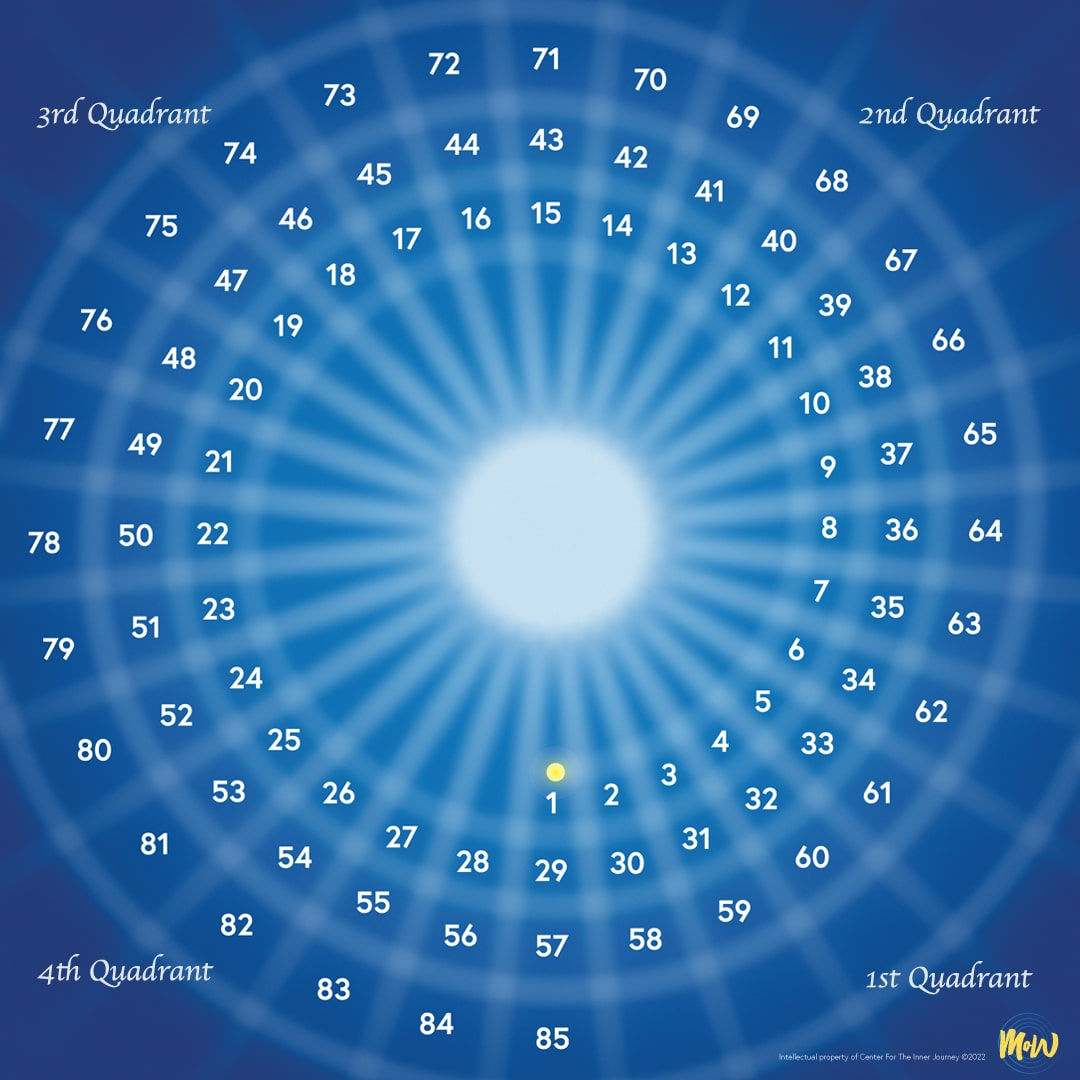On the image click on the number that represents your chronological age.Here is how it works
For instance, if your age is 60, your corresponding ages are 32 and 4. If your age is 33 your corresponding age is 5.

Next you will see how this recording is used by looking at some examples of actual MoW worksheets called The Table for Refection (TFR).

Here is how it works
For instance, if your age is 66, your corresponding ages are 38 and 10. If your age is 39 your corresponding age is 11.

Next you will see how this recording is used by looking at some examples of actual MoW worksheets called The Table for Refection (TFR).